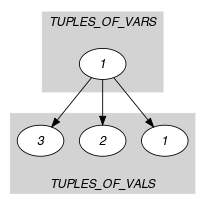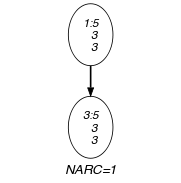## 5.181. in_relation

Origin

Constraint explicitly defined by tuples of values.

Constraint

$\mathrm{𝚒𝚗}_\mathrm{𝚛𝚎𝚕𝚊𝚝𝚒𝚘𝚗}\left(\mathrm{𝚅𝙰𝚁𝙸𝙰𝙱𝙻𝙴𝚂},\mathrm{𝚃𝚄𝙿𝙻𝙴𝚂}_\mathrm{𝙾𝙵}_\mathrm{𝚅𝙰𝙻𝚂}\right)$

Synonyms

$\mathrm{𝚌𝚊𝚜𝚎}$, $\mathrm{𝚎𝚡𝚝𝚎𝚗𝚜𝚒𝚘𝚗}$, $\mathrm{𝚎𝚡𝚝𝚎𝚗𝚜𝚒𝚘𝚗𝚊𝚕}$, $\mathrm{𝚎𝚡𝚝𝚎𝚗𝚜𝚒𝚘𝚗𝚊𝚕}_\mathrm{𝚜𝚞𝚙𝚙𝚘𝚛𝚝}$, $\mathrm{𝚎𝚡𝚝𝚎𝚗𝚜𝚒𝚘𝚗𝚊𝚕}_\mathrm{𝚜𝚞𝚙𝚙𝚘𝚛𝚝𝚟𝚊}$, $\mathrm{𝚎𝚡𝚝𝚎𝚗𝚜𝚒𝚘𝚗𝚊𝚕}_\mathrm{𝚜𝚞𝚙𝚙𝚘𝚛𝚝𝚖𝚍𝚍}$, $\mathrm{𝚎𝚡𝚝𝚎𝚗𝚜𝚒𝚘𝚗𝚊𝚕}_\mathrm{𝚜𝚞𝚙𝚙𝚘𝚛𝚝𝚜𝚝𝚛}$, $\mathrm{𝚏𝚎𝚊𝚜𝚝𝚞𝚙𝚕𝚎𝚊𝚌}$, $\mathrm{𝚝𝚊𝚋𝚕𝚎}$.

Types
 $\mathrm{𝚃𝚄𝙿𝙻𝙴}_\mathrm{𝙾𝙵}_\mathrm{𝚅𝙰𝚁𝚂}$ $\mathrm{𝚌𝚘𝚕𝚕𝚎𝚌𝚝𝚒𝚘𝚗}\left(\mathrm{𝚟𝚊𝚛}-\mathrm{𝚍𝚟𝚊𝚛}\right)$ $\mathrm{𝚃𝚄𝙿𝙻𝙴}_\mathrm{𝙾𝙵}_\mathrm{𝚅𝙰𝙻𝚂}$ $\mathrm{𝚌𝚘𝚕𝚕𝚎𝚌𝚝𝚒𝚘𝚗}\left(\mathrm{𝚟𝚊𝚕}-\mathrm{𝚒𝚗𝚝}\right)$
Arguments
 $\mathrm{𝚅𝙰𝚁𝙸𝙰𝙱𝙻𝙴𝚂}$ $\mathrm{𝚃𝚄𝙿𝙻𝙴}_\mathrm{𝙾𝙵}_\mathrm{𝚅𝙰𝚁𝚂}$ $\mathrm{𝚃𝚄𝙿𝙻𝙴𝚂}_\mathrm{𝙾𝙵}_\mathrm{𝚅𝙰𝙻𝚂}$ $\mathrm{𝚌𝚘𝚕𝚕𝚎𝚌𝚝𝚒𝚘𝚗}\left(\mathrm{𝚝𝚞𝚙𝚕𝚎}-\mathrm{𝚃𝚄𝙿𝙻𝙴}_\mathrm{𝙾𝙵}_\mathrm{𝚅𝙰𝙻𝚂}\right)$
Restrictions
 $\mathrm{𝚛𝚎𝚚𝚞𝚒𝚛𝚎𝚍}$$\left(\mathrm{𝚃𝚄𝙿𝙻𝙴}_\mathrm{𝙾𝙵}_\mathrm{𝚅𝙰𝚁𝚂},\mathrm{𝚟𝚊𝚛}\right)$ $|\mathrm{𝚃𝚄𝙿𝙻𝙴}_\mathrm{𝙾𝙵}_\mathrm{𝚅𝙰𝚁𝚂}|\ge 1$ $|\mathrm{𝚃𝚄𝙿𝙻𝙴}_\mathrm{𝙾𝙵}_\mathrm{𝚅𝙰𝙻𝚂}|\ge 1$ $|\mathrm{𝚃𝚄𝙿𝙻𝙴}_\mathrm{𝙾𝙵}_\mathrm{𝚅𝙰𝙻𝚂}|=|\mathrm{𝚅𝙰𝚁𝙸𝙰𝙱𝙻𝙴𝚂}|$ $\mathrm{𝚛𝚎𝚚𝚞𝚒𝚛𝚎𝚍}$$\left(\mathrm{𝚃𝚄𝙿𝙻𝙴}_\mathrm{𝙾𝙵}_\mathrm{𝚅𝙰𝙻𝚂},\mathrm{𝚟𝚊𝚕}\right)$ $\mathrm{𝚛𝚎𝚚𝚞𝚒𝚛𝚎𝚍}$$\left(\mathrm{𝚃𝚄𝙿𝙻𝙴𝚂}_\mathrm{𝙾𝙵}_\mathrm{𝚅𝙰𝙻𝚂},\mathrm{𝚝𝚞𝚙𝚕𝚎}\right)$
Purpose

Enforce the tuple of variables $\mathrm{𝚅𝙰𝚁𝙸𝙰𝙱𝙻𝙴𝚂}$ to take its value out of a set of tuples of values $\mathrm{𝚃𝚄𝙿𝙻𝙴𝚂}_\mathrm{𝙾𝙵}_\mathrm{𝚅𝙰𝙻𝚂}$. The value of a tuple of variables $〈{V}_{1},{V}_{2},\cdots ,{V}_{n}〉$ is a tuple of values $〈{U}_{1},{U}_{2},\cdots ,{U}_{n}〉$ if and only if ${V}_{1}={U}_{1}\wedge {V}_{2}={U}_{2}\wedge \cdots \wedge {V}_{n}={U}_{n}$.

Example
$\left(\begin{array}{c}〈5,3,3〉,\hfill \\ 〈\mathrm{𝚝𝚞𝚙𝚕𝚎}-〈5,2,3〉,\mathrm{𝚝𝚞𝚙𝚕𝚎}-〈5,2,6〉,\mathrm{𝚝𝚞𝚙𝚕𝚎}-〈5,3,3〉〉\hfill \end{array}\right)$

The $\mathrm{𝚒𝚗}_\mathrm{𝚛𝚎𝚕𝚊𝚝𝚒𝚘𝚗}$ constraint holds since its first argument $〈5,3,3〉$ corresponds to the third item of the collection of tuples $\mathrm{𝚃𝚄𝙿𝙻𝙴𝚂}_\mathrm{𝙾𝙵}_\mathrm{𝚅𝙰𝙻𝚂}$.

Typical
$|\mathrm{𝚃𝚄𝙿𝙻𝙴}_\mathrm{𝙾𝙵}_\mathrm{𝚅𝙰𝚁𝚂}|>1$
Symmetries
• Items of $\mathrm{𝚃𝚄𝙿𝙻𝙴𝚂}_\mathrm{𝙾𝙵}_\mathrm{𝚅𝙰𝙻𝚂}$ are permutable.

• Items of $\mathrm{𝚅𝙰𝚁𝙸𝙰𝙱𝙻𝙴𝚂}$ and $\mathrm{𝚃𝚄𝙿𝙻𝙴𝚂}_\mathrm{𝙾𝙵}_\mathrm{𝚅𝙰𝙻𝚂}.\mathrm{𝚝𝚞𝚙𝚕𝚎}$ are permutable (same permutation used).

• All occurrences of two distinct tuples of values in $\mathrm{𝚅𝙰𝚁𝙸𝙰𝙱𝙻𝙴𝚂}$ or $\mathrm{𝚃𝚄𝙿𝙻𝙴𝚂}_\mathrm{𝙾𝙵}_\mathrm{𝚅𝙰𝙻𝚂}.\mathrm{𝚝𝚞𝚙𝚕𝚎}$ can be swapped; all occurrences of a tuple of values in $\mathrm{𝚅𝙰𝚁𝙸𝙰𝙱𝙻𝙴𝚂}$ or $\mathrm{𝚃𝚄𝙿𝙻𝙴𝚂}_\mathrm{𝙾𝙵}_\mathrm{𝚅𝙰𝙻𝚂}.\mathrm{𝚝𝚞𝚙𝚕𝚎}$ can be renamed to any unused tuple of values.

Arg. properties

Extensible wrt. $\mathrm{𝚃𝚄𝙿𝙻𝙴𝚂}_\mathrm{𝙾𝙵}_\mathrm{𝚅𝙰𝙻𝚂}$.

Usage

Quite often some constraints cannot be easily expressed, neither by a formula, nor by a regular pattern. In this case one has to define the constraint by specifying in extension the combinations of allowed values.

Remark

The $\mathrm{𝚒𝚗}_\mathrm{𝚛𝚎𝚕𝚊𝚝𝚒𝚘𝚗}$ constraint is called $\mathrm{𝚎𝚡𝚝𝚎𝚗𝚜𝚒𝚘𝚗𝚊𝚕}_\mathrm{𝚜𝚞𝚙𝚙𝚘𝚛𝚝}$ in JaCoP (http://www.jacop.eu/). Within SICStus Prolog the constraint can be applied to more than a single tuple of variables and is called $\mathrm{𝚝𝚊𝚋𝚕𝚎}$. Within [BourdaisGalinierPesant03] this constraint is called $\mathrm{𝚎𝚡𝚝𝚎𝚗𝚜𝚒𝚘𝚗}$.

The $\mathrm{𝚒𝚗}_\mathrm{𝚛𝚎𝚕𝚊𝚝𝚒𝚘𝚗}$ constraint is called $\mathrm{𝚝𝚊𝚋𝚕𝚎}$ in MiniZinc (http://www.minizinc.org/).

Systems
Used in

cost variant: $\mathrm{𝚌𝚘𝚗𝚍}_\mathrm{𝚕𝚎𝚡}_\mathrm{𝚌𝚘𝚜𝚝}$ ($\mathrm{𝙲𝙾𝚂𝚃}$ parameter added).

Keywords
Derived Collection
$\mathrm{𝚌𝚘𝚕}\left(\begin{array}{c}\mathrm{𝚃𝚄𝙿𝙻𝙴𝚂}_\mathrm{𝙾𝙵}_\mathrm{𝚅𝙰𝚁𝚂}-\mathrm{𝚌𝚘𝚕𝚕𝚎𝚌𝚝𝚒𝚘𝚗}\left(\mathrm{𝚟𝚎𝚌}-\mathrm{𝚃𝚄𝙿𝙻𝙴}_\mathrm{𝙾𝙵}_\mathrm{𝚅𝙰𝚁𝚂}\right),\hfill \\ \mathrm{𝚒𝚝𝚎𝚖}\left(\mathrm{𝚟𝚎𝚌}-\mathrm{𝚅𝙰𝚁𝙸𝙰𝙱𝙻𝙴𝚂}\right)\right]\hfill \end{array}\right)$
Arc input(s)

$\mathrm{𝚃𝚄𝙿𝙻𝙴𝚂}_\mathrm{𝙾𝙵}_\mathrm{𝚅𝙰𝚁𝚂}$ $\mathrm{𝚃𝚄𝙿𝙻𝙴𝚂}_\mathrm{𝙾𝙵}_\mathrm{𝚅𝙰𝙻𝚂}$

Arc generator
$\mathrm{𝑃𝑅𝑂𝐷𝑈𝐶𝑇}$$↦\mathrm{𝚌𝚘𝚕𝚕𝚎𝚌𝚝𝚒𝚘𝚗}\left(\mathrm{𝚝𝚞𝚙𝚕𝚎𝚜}_\mathrm{𝚘𝚏}_\mathrm{𝚟𝚊𝚛𝚜},\mathrm{𝚝𝚞𝚙𝚕𝚎𝚜}_\mathrm{𝚘𝚏}_\mathrm{𝚟𝚊𝚕𝚜}\right)$

Arc arity
Arc constraint(s)
$\mathrm{𝚟𝚎𝚌}_\mathrm{𝚎𝚚}_\mathrm{𝚝𝚞𝚙𝚕𝚎}$$\left(\mathrm{𝚝𝚞𝚙𝚕𝚎𝚜}_\mathrm{𝚘𝚏}_\mathrm{𝚟𝚊𝚛𝚜}.\mathrm{𝚟𝚎𝚌},\mathrm{𝚝𝚞𝚙𝚕𝚎𝚜}_\mathrm{𝚘𝚏}_\mathrm{𝚟𝚊𝚕𝚜}.\mathrm{𝚝𝚞𝚙𝚕𝚎}\right)$
Graph property(ies)
$\mathrm{𝐍𝐀𝐑𝐂}$$\ge 1$

Graph model

Parts (A) and (B) of Figure 5.181.1 respectively show the initial and final graph associated with the Example slot. Since we use the $\mathrm{𝐍𝐀𝐑𝐂}$ graph property, the unique arc of the final graph is stressed in bold.

##### Figure 5.181.1. Initial and final graph of the $\mathrm{𝚒𝚗}_\mathrm{𝚛𝚎𝚕𝚊𝚝𝚒𝚘𝚗}$ constraint(a) (b)